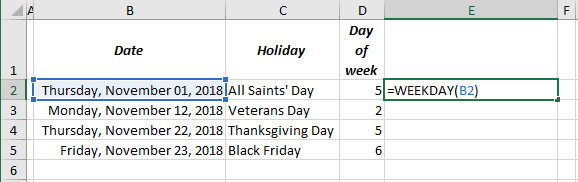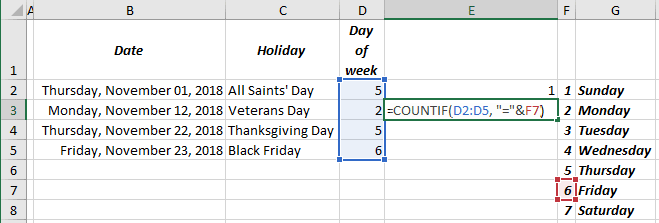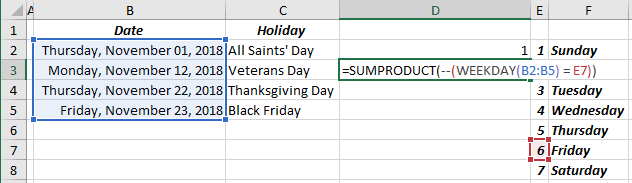# How to count days of week in the range of dates

Excel
From time to time, you need to count days of week in some date range or between two dates (see How to count days of week between two dates). Excel proposes different formulas to work with data. It is easy to create the formula you need for this task:

The formula WEEKDAY returns a number between 1 and 7 that corresponds to a particular day of the week. With default settings, 1 = Sunday, 2 = Monday, 3 = Tuesday, 4 = Wednesday, 5 = Thursday, 6 = Friday and 7 = Saturday.

To count days of week for the date range, for example, to count Fridays in the November holidays (range B2:B5), do one of the following:

## To use the COUNTIF formula

1.   Add new column (or row) for the range and insert the formula:

= WEEKDAY (<date>):2.   Use the formula COUNTIF (<data range>, criteria) with the following parameters:

= COUNTIF (D2:D5, "=6"):

or

= COUNTIF (D2:D5, "="&F6):## To use the SUMPRODUCT formula

This formula is more complex, but doesn’t need any additional calculation:

= SUMPRODUCT (-- (WEEKDAY (B2:B5) = 6))

or

= SUMPRODUCT (-- (WEEKDAY (B2:B5) = E7)):Whenever you put -- (two hyphens) in the front of the parenthesis, it changes all the values TRUE / FALSE in the array in the parenthesis to the values 1 / 0. So:

• the formula (WEEKDAY (<date range>) = 6) returns the array of TRUE and FALSE,
• (-- <array>) returns array of 1 and 0 instead of TRUE and FALSE,
• SUMPRODUCT just sums all values of an array.

See also this tip in French: Comment compter les jours de la semaine dans la plage de dates.